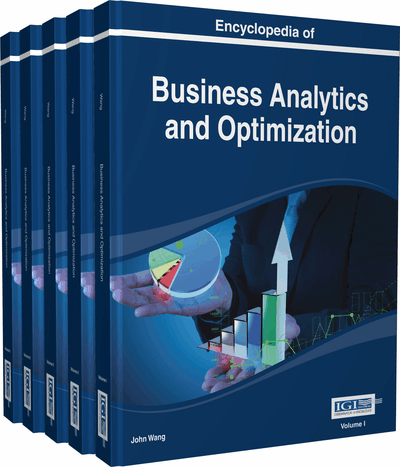# Data Mining Tools: Formal Concept Analysis and Rough Sets

Sanjiv K. Bhatia (University of Missouri, USA) and Jitender S. Deogun (University of Nebraska, USA)
DOI: 10.4018/978-1-4666-5202-6.ch060

Top

## Main Focus Of The Chapter

### Formal Concept Analysis and Data Mining

Formal concept analysis (FCA) can be used to derive conceptual structures, analyze complex structures, and discover data dependencies (Wille, 1989; Wille, 2005). FCA is useful in data mining in two ways. First, it provides tools for formal representation of knowledge in an efficient manner. Second, it helps to formalize the conceptual knowledge discovery for different data mining tasks. FCA is increasingly applied in conceptual clustering, data analysis, information retrieval, knowledge discovery, and ontology engineering. Though different from first order logic, FCA emphasizes inter-subjective communication and argumentation. FCA also facilitates importation of the notion of a concept into the modeling of knowledge discovery in databases (KDD).

Formal concept analysis is based on the notions of formal context and formal concept. A formal context is a binary relation between a set of objects and a set of attributes. A formal context provides logic representation of a data set and is used to extract formal concepts.

A formal concept is a pair of intent and extent (Saquer & Deogun, 1999). Intent is a set of features possessed by each object. The extent represents the set of all objects that belong to the concept. These objects share the features from intent. Given a set of features in intent, we can find objects that share the set or subset of features that are shared by the candidates in the extent. There may exist some indiscernible objects in the extent; such objects can be classified using concept learning from formal concept analysis.

### Formal Context

A formal context is defined by a triplet (O,A,R), where O and A are two finite and nonempty sets, namely the object set and the attribute set. The relationships between objects and attributes are described by a binary relation R between O and A, which is a subset of the Cartesian product O×A. If an object Ox possesses an attribute Ay, we denote it as (Ox,Ay)∈R, or OxRAy.

Based on the definition of formal context, we know that an object OxO has a set of attributes:

and an attribute Ay is possessed by the set of objects:

To perform FCA, we first define a set-theoretic operator “*” to associate the subset of objects and attributes mutually in a formal context (O,A,R).

This shows that the “*” operator associates a subset of attributes X* to the subset of objects X. Similarly, for any subset of attributes, we can associate a subset of objectsas follows:

(1)(2)(3)(4)

A pair of mappings is called a Galois connection if it satisfies (1) and (2), and hence (3). By definition,is the set of attributes possessed by Ox, andis the set of objects having attributes Ay. For a set of objects X, X* is the maximal set of attributes shared by all objects in X. Similarly, for a set of attributes Y, Y* is the maximal set of objects that have all attributes in Y (Yao & Chen, 2006).

## Key Terms in this Chapter

Association Rules: Identification of statistically related attributes in data.

Bayesian Classification: Classification based on naïve Bayesian probabilistic analysis.

Clustering: Organization of data in some semantically meaningful way such that each cluster contains related data while the unrelated data are assigned to different clusters. The clusters may not be predefined.

Reduct: A structural method to discover data dependencies in rough set theory.

Decision Tree: A tool to help make decisions based on a set of rules that help to navigate the tree along its branches.

Induction-Based Learning: Learning by observation of different objects or data; building general concepts by observing a set of instances.

Dimensionality Reduction: Consolidating the range of a set of attributes for efficient analysis.

Similarity Measure: A tool to quantify the similarity between different objects.

Conceptual Clustering: Clustering of data based on concepts that may define related terms as a thesaurus.

## Complete Chapter List

Search this Book:
Reset